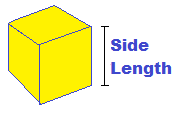Math isn't easy for everyone,
CalcuNation is.

# Cube Volume Calculator

## Find the volume of a cube with this Cube Volume Calculator.Side Length:
Fill in the length of one of the cube sides to determine volume.

### How do you find the volume of a cube?

To find the volume, use this cube volume formula:
Cube Volume = Side Length3

Example: The volume of a cube with a side length of 3 inches is:
Cube Volume = 33
Calculated out this gives a volume of 27 Cubic Inches.